# Structures in C programming with examples

It is possible to combine data items of various types using the structure, which is a user-defined data type. In other words, the structure is nothing more than a collection of variables that are grouped together under a single name, which is referred to as a "aggregate data type."

## Define a Structure in C

To define a structure in C, all you have to do is use the "struct" keyword. The following is the general form that must be used when defining a structure in C:

```struct struct_name
{
type member_name1;
type member_name2;
type member_name3;
.
.
.
type member_nameN;
}structure_variable_name;```

Here "struct" is a keyword used in declaring a structure in C. Then struct_name is the name of the structure. Then type can be any valid data type. And then, member_name1, member_name2, member_name3, and member_nameN are the names of the structure members. Finally, structure_variable_name is the name of the structure variable that is used to access a member of this structure using the dot operator. You can also declare structure_variable_name later. You can also use this general form.

```struct [structure tag]
{
member definition;
member definition;
member definition;
...
...
member definition;
} [structure variables];```

Let's look at this declaration to understand how to define a structure in the C language:

```struct address
{
char name;
char street;
char city;
char state;
unsigned long int zip;

Here we define a structure type called address and declare a variable named addr_var of that type. When a structure variable (addr_var here) is declared, then the compiler automatically allocates sufficient memory to accommodate all of its members.

## Access Structure Members in C

To access individual members of a structure in C,  simply use the dot (.) operator. Here is the general form to access structure members in C:

`object_name.member_name;`

Let's look at the following code fragment, demonstrating how to access structure members:

`addr_var.zip = 20500;`

And to print the ZIP code on the screen, follow this statement:

`printf("%lu", addr_var.zip);`

The above statement shows the zip code from the structure variable addr_var's zip member.

## Structure Assignments in C

In C, one structure can also be assigned to another structure of the same type. Using a single assignment statement, the information contained in one structure can be assigned to another structure of the same type. The following is the standard format for assigning one structure to another of the same type:

```struct struct_name
{
type member_name1;
type member_name2;
type member_name3;
.
.
.
type member_nameN;
} struct_variable1, struct_variable2;

struct_variable1 = struct_variable2;```

Here is an example program demonstrating structure assignment:

```#include <stdio.h>

struct st
{
int x;
int y;
int z;
char ch;
char chs;
} st1, st2;

int main()
{
gets(st1.chs);
printf("Enter any three numbers: ");
scanf("%d%d%d", &st1.x, &st1.y, &st1.z);
printf("Press 'y' and then hit the ENTER key to see the sum: ");
fflush(stdin);
scanf("%c", &st1.ch);

st2 = st1;              // assign one structure to another

if (st2.ch == 'y' || st2.ch == 'Y')
{
printf("%s, the sum of three numbers is %d.", st2.chs, st2.x + st2.y + st2.z);
return 0;
}

return 0;
}```

The following snapshot shows the initial output produced by the above program: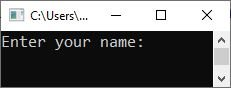Now enter your name, "William," and press the ENTER key. Then enter three numbers in the following order: enter the first number, say 10, and press the ENTER key, then enter the second number, say 20, and press the ENTER key, and finally type the third number, say 30, and press the ENTER key to get the following output: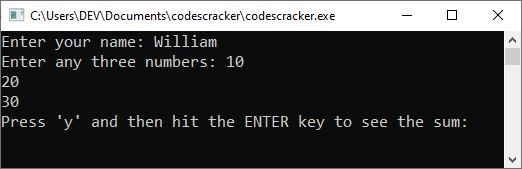Now, type "y" and press the ENTER key to see the sum of the three numbers you entered on the output console, as shown in the screenshot below: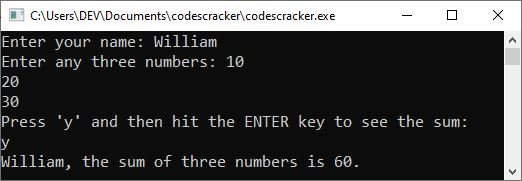As you can see, this program gets the value entered by the user and stores it in the structure members accessed by the first structure (the structure variable), and then we assign it to another structure (the structure variable). So that all the members' values in the first structure can be accessed using the second structure (structure variables) as well.

The method "fflush()" is used to flush the output buffer.

## Arrays of Structures in C

Typically, structures are arrayed. To declare an array of structures, you must first define a structure, followed by the declaration of an array variable of that type. Here is an instance of declaring a 10-element structure array of type st:

`struct st st_var;`

The preceding statement generates ten sets of variables organized in accordance with the st structure.

To gain access to a particular structure, simply index the array's name. Here is an example of accessing the structure 4 member zip:

`st_var.zip;`

Indexing always begins with 0, so index number 0 refers to the first element, index number 1 to the second element, and index number 3 to the fourth element. Here's an example of how to assign a value to structure 4's member zip:

`st_var.zip = 20500;`

Here is an example of printing the value of member zip in structure 4:

`printf("%lu", st_var.zip);`

Here is an example, assigning "A" to the first character of name in the fourth structure of st_var:

`st_var.name = 'A';`

Let's look at the following example for a complete understanding of the concept of arrays of structures in the C language:

```#include <stdio.h>

struct st
{
char name;
char street;
char city;
char state;
unsigned long int zip;
};

int main()
{
struct st st_var;
printf("Enter name: ");
gets(st_var.name);
printf("Enter street: ");
gets(st_var.street);
printf("Enter city: ");
gets(st_var.city);
printf("Enter state: ");
gets(st_var.state);
scanf("%lu", &st_var.zip);

printf("\nName\tStreet\t\t\tCity\t\tState\tZip Code\n");
printf("%s\t%s\t\t%s\t%s\t%lu \n", st_var.name, st_var.street, st_var.city, st_var.state, st_var.zip);

return 0;
}```

The following snapshot shows the sample run after providing the following inputs:

• "William" as the name.
• "Hollywood Boulevard" as the street.
• "Los Angeles" as the city.
• "California" as the state.
• "90027" as the zip code.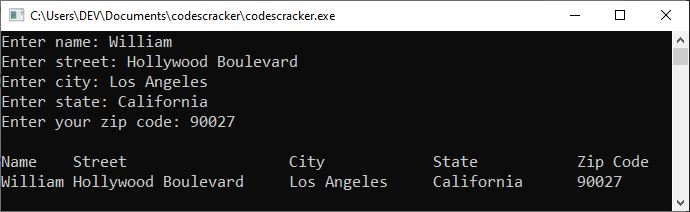## Pass structures to functions in C

In C, it is permissible to pass structure members or entire structures to functions. Whenever you pass a structure member to a function, you are passing the member's value to the function. Consider the following structure declaration:

```struct st
{
int num;
char ch;
char str;
} st_var;```

Here is an example of passing each member to a function:

```function(st_var.num);      // passes the integer value of "num"
function(st_var.ch);       // passes the character value of "ch"
function(st_var.str);   // passes the character value of "str"
function(st_var.str);      // passes the address of the string "str"```

Here is another example of passing the address of each member to a function:

```function(&st_var.num);     // passes the address of the integer "num"
function(&st_var.ch);      // passes the address of the character "ch"
function(&st_var.str);  // passes the address of the character "str"
function(st_var.str);      // passes the address of the string "str"```

When a structure is passed as an argument to a function in this instance, the entire structure is passed using the standard call-by-value method. Here is an example of passing an entire structure to a function:

```#include <stdio.h>

struct st
{
int num1;
int num2;
char ch;
char name;
};

void fun(struct st stinf);

int main()
{
struct st stoutf;

gets(stoutf.name);
printf("Enter any two numbers: ");
scanf("%d %d", &stoutf.num1, &stoutf.num2);
printf("Type 'y' and hit the ENTER key to see the sum.\n");
fflush(stdin);
scanf("%c", &stoutf.ch);
if (stoutf.ch == 'y' || stoutf.ch == 'Y')
{
printf("\n");
fun(stoutf);
return 0;
}

return 0;
}

void fun(struct st stinf)
{
printf("%s, the sum of two numbers is %d \n", stinf.name, stinf.num1 + stinf.num2);
}```

The following snapshot shows the sample run.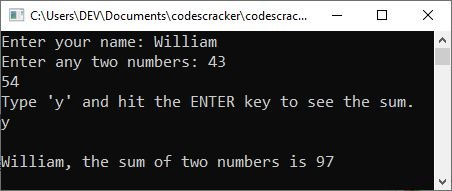## Structure Pointers in C

In the C programming language, pointers to structures are permitted. Here is the general form of a structure pointer declaration in C:

`struct st *st_pointer;`

To determine the address of any structure variable, prefix the structure's name with the & operator. Consider the following structure declaration:

```struct stc
{
int num;
char ch;
char str;
} stc_var;

struct stc *ptr;      // declared a structure pointer```

Now the following code fragment simply places the address of the structure stc_var into the pointer ptr:

`ptr = &stc_var;`

And you must use the "->" operator to access the members of a structure using a pointer to that structure. Here is an illustration using the "num" field:

`ptr->num`

Here is an illustration of structure pointers in the C programming language. Before I include the program, I'd like to point out that it continues to print the time.

```#include <stdio.h>

#define DELAY 128000
#define TEMP_DELAY 59

struct st_time
{
int hours;
int minutes;
int seconds;
};

void display(struct st_time *time);
void update(struct st_time *time);
void delay(void);

int main()
{
struct st_time systime;

systime.hours = 0;
systime.minutes = 0;
systime.seconds = 0;

for (;;)
{
update(&systime);
display(&systime);
}

return 0;
}

void update(struct st_time *time)
{
time->seconds++;
if (time->seconds == 60)
{
time->seconds = 0;
time->minutes++;
}

if (time->minutes == 60)
{
time->minutes = 0;
time->hours++;
}

if (time->hours == 24)
time->hours = 0;

delay();
}

void display(struct st_time *time)
{
printf("%02d:", time->hours);
printf("%02d:", time->minutes);
printf("%02d\n", time->seconds);
}

void delay(void)
{
long int time, temp;

for (temp = 0; temp < TEMP_DELAY; temp++)
for (time = 1; time < DELAY; time++) ;
}```

Here is a sample run of this C program. This program continues to display the time starting from 00:00:00.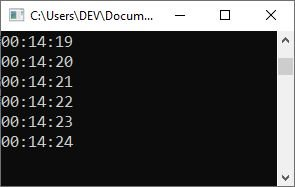C Online Test

« Previous Tutorial Next Tutorial »